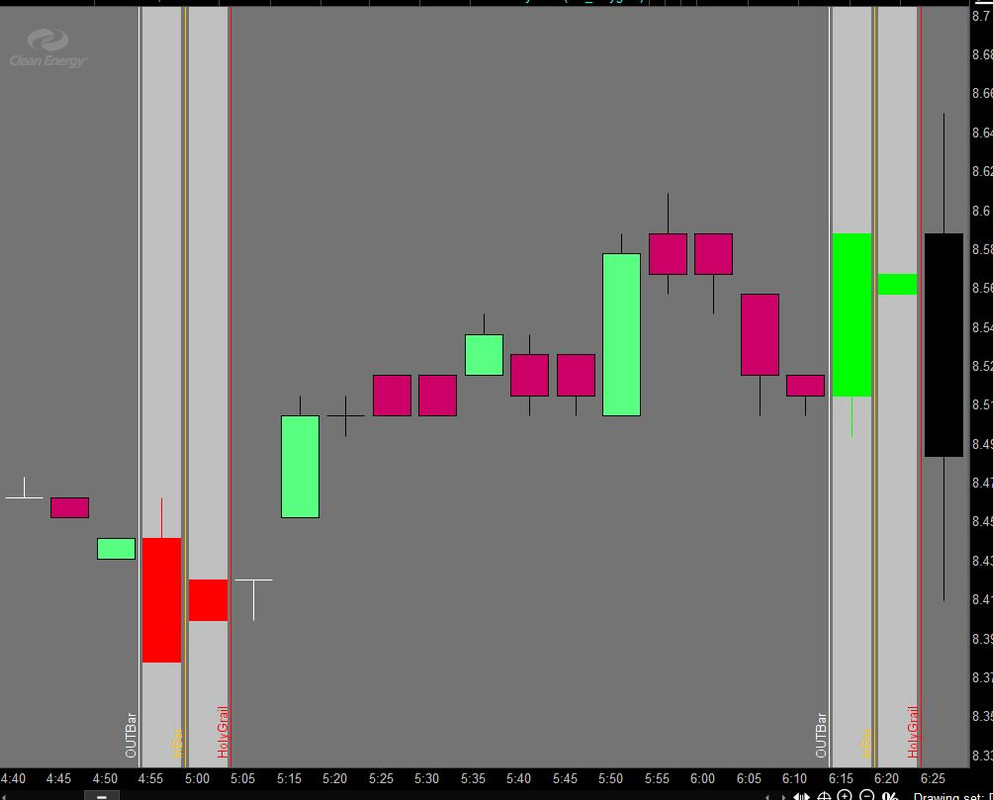# adding a cloud between two bars?

##### New member
i am trying to add a cloud between the two vertical lines. I am able to put the vertical lines but not the cloud. How can I do that?

plot inbar = low > low and high < high;
plot outbar = low < low and high > high;
plot holygrail = outbar and inbar;

AssignPriceColor(if (inbar and !holygrail) then Color.YELLOW else Color.CURRENT);
AssignPriceColor(if (outbar and !holygrail) then Color.BLACK else Color.CURRENT);

#### halcyonguy

##### Active member
VIP
i am trying to add a cloud between the two vertical lines. I am able to put the vertical lines but not the cloud. How can I do that?

plot inbar = low > low and high < high;
plot outbar = low < low and high > high;
plot holygrail = outbar and inbar;

AssignPriceColor(if (inbar and !holygrail) then Color.YELLOW else Color.CURRENT);
AssignPriceColor(if (outbar and !holygrail) then Color.BLACK else Color.CURRENT);

you need to define 2 price levels, for a lower and upper boundry , for the cloud.

to define a time to enable them, could be something like this
Code:
``````def x = (holygrail or holygrail or holygrail[-1] );

def cloudtop = if x then close + 1 else double.nan;
def cloudbot = if x then close - 1 else double.nan;

addcloud( cloudtop , cloudbot, color.gray, color.gray);``````

•#### SleepyZ

##### Active member
i am trying to add a cloud between the two vertical lines. I am able to put the vertical lines but not the cloud. How can I do that?

plot inbar = low > low and high < high;
plot outbar = low < low and high > high;
plot holygrail = outbar and inbar;

AssignPriceColor(if (inbar and !holygrail) then Color.YELLOW else Color.CURRENT);
AssignPriceColor(if (outbar and !holygrail) then Color.BLACK else Color.CURRENT);

TOS's 'addcloud' function does not work very well drawing clouds between vertical lines as the clouds do not align within the vertical lines. A workaround is to use the 'unsupported', but available to use 'addchart' function.Ruby:
``````plot inbar = low > low and high < high;
plot outbar = low < low and high > high;
plot holygrail = outbar and inbar;

AssignPriceColor(if (inbar and !holygrail) then Color.YELLOW else Color.CURRENT);
AssignPriceColor(if (outbar and !holygrail) then Color.BLACK else Color.CURRENT);

#########################
#Workaround Cloud between vertical lines using addchart()

def h = Double.POSITIVE_INFINITY;
def l = Double.NEGATIVE_INFINITY;
def xoutbar  = if holygrail[-1] and outbar then 1 else 0;
def xinbar   = if holygrail     and inbar  then 1 else 0;
def xboth    = if xoutbar == 1
then 1 else
if xboth == 1 and xinbar == 1
then 1 else 0;

input cloud = {default out_in, out_holygrail, in_holygrail, NONE};

def xH =     if cloud == cloud.NONE
then Double.NaN else
if cloud == cloud.out_in        and xoutbar  == 1  or
cloud == cloud.out_holygrail and xboth or
cloud == cloud.in_holygrail  and xinbar   == 1
then h else Double.NaN;
def xL =     if cloud == cloud.NONE
then Double.NaN else
if cloud == cloud.out_in        and xoutbar == 1 or
cloud == cloud.out_holygrail and xboth or
cloud == cloud.in_holygrail  and xinbar  == 1
then l else Double.NaN;

AddChart(high = xH, low = xL, open = xH, close = xL, type = ChartType.CANDLE, growColor = Color.LIGHT_GRAY);

##########################
#Display candles hidden by above workaround
def rh =     if cloud == cloud.NONE
then Double.NaN else
if cloud == cloud.out_in        and xoutbar == 1 or
cloud == cloud.out_holygrail and xboth or
cloud == cloud.in_holygrail  and xinbar  == 1
then high else Double.NaN;

def rl =     if cloud == cloud.NONE
then Double.NaN else
if cloud == cloud.out_in        and xoutbar == 1 or
cloud == cloud.out_holygrail and xboth or
cloud == cloud.in_holygrail  and xinbar  == 1
then low else Double.NaN;
def rhg = if close >= open then rh else Double.NaN;
AddChart(high = rhg, low = rl, open = close, close = open, growColor = Color.GREEN, type = ChartType.CANDLE);

def rhr = if close < open then rh else Double.NaN;
AddChart(high = rhr, low = rl, open = open, close = close, growColor = Color.RED, type = ChartType.CANDLE);``````

•